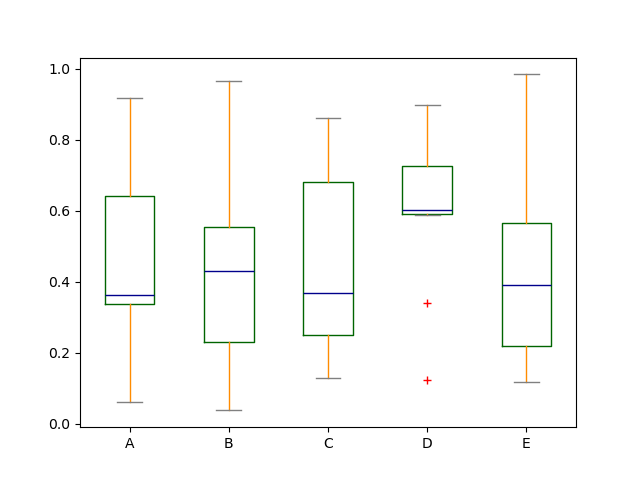All Courses

# How to plot box plot using pandas?

##### By Albert, 2 years ago

Plot the customize box plot using pandas

Box plot
Pandas
##### Poornima

Boxplot can be drawn calling Series.plot.box() and DataFrame.plot.box(), or DataFrame.boxplot() to visualize the distribution of values within each column.

For instance, here is a boxplot representing five trials of 10 observations of a uniform random variable on [0,1).

```df = pd.DataFrame(np.random.rand(10, 5), columns=["A", "B", "C", "D", "E"])

df.plot.box()
```Boxplot can be colorized by passing color keyword. You can pass a dict whose keys are boxes, whiskers, medians and caps. If some keys are missing in the dict, default colors are used for the corresponding artists. Also, boxplot has sym keyword to specify fliers style.

```color = {
"boxes": "DarkGreen",
"whiskers": "DarkOrange",
"medians": "DarkBlue",
"caps": "Gray",
}

df.plot.box(color=color, sym="r+");
```#### Why You Should Learn Data Science in 2023?Jun 8th (7:00 PM) 289 Registered
More webinars

#### Related Discussions

Running random forest algorithm with one variable

View More